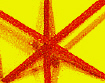Representation of the Spectral Line Structure of the H Atom - 1987Home Manifesto & First Exhibition Phase 1 - Some Artistic Works Other Publications Lecture at Whitechapel Gallery Artistic Represent-ation of Hydrogen Atom Quantum Realism in the Nineties ContactThis  lecture was given at the  WhiteChapel Art Gallery Lecture Theatre (London) on 5th April 1987. ( The text was published in the Constructivist Forum, Issue No.6 -London-1987).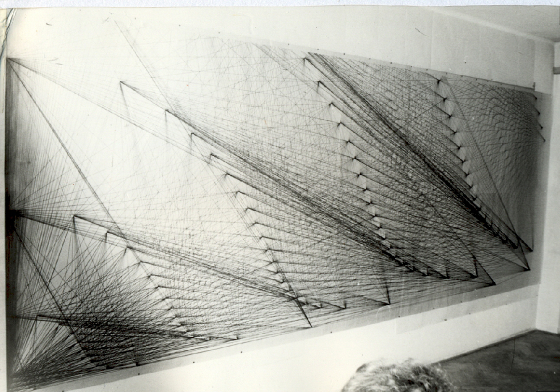Representation of the spectral line structure of the H Atom - 1987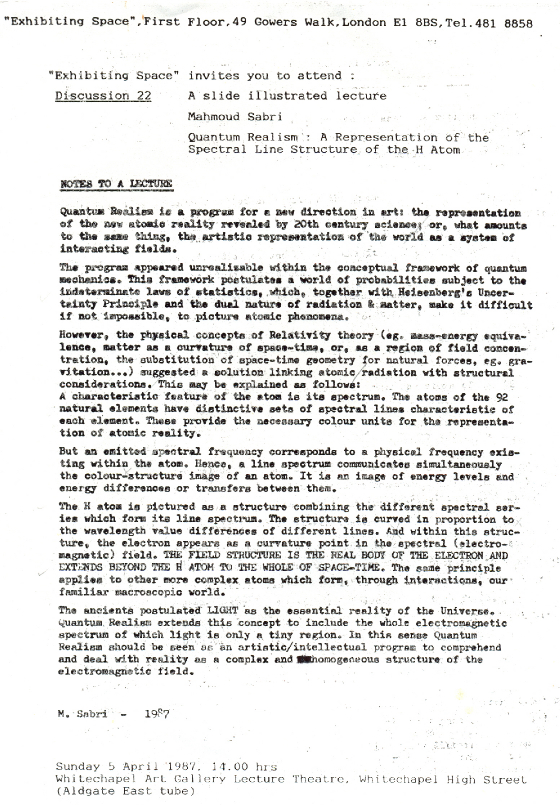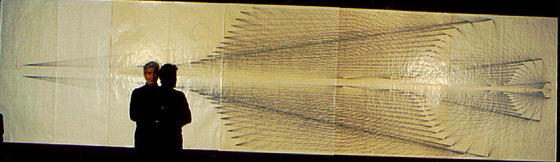M.Sabri in front of a representation of the spectral lines of the H Atom - 1987

A REPRESENTATION OF THE SPECTRAL LINE STRUCTURE. OF THE H ATOM

M.Sabri

____________________________________________________________________

In 1971 I defined QUANTUM REALISM (Exhibition Program, Prague, 26/10/71 – 14/11/71) as a 'graphic experiment synthesizing 2 basic concepts of our age:

(2) MASS-ENERGY EQUIVAIENCE: E = M C2 (Einstein).

The synthesis suggested a picture of the world as a SYSTEM OF ENERGY- PROCESSES. Such a picture however could only be plastically renderedif the correct units are employed. These have to be derived from nature's ELEMENTARY ie. ATOMIC PROCESSES.

1

I "started with the CHEMICAL PROCESSES through which macroscopic phenomena are formed by the interactions/combinations of different atoms. A charac­teristic feature of an atom is its EMISSION LINE SPECTRUM, ie. the series of coloured lines emitted by aD atom in a state of excitation. The atoms of each of the 92 natural elements have well-defined sets of such spectral lines. These constitute the visible quantities by which the otherwise invisible atoms are recognized. Atomic matter therefore finds its distinc­tive graphic expression in the pure colour of its spectral radiation.

The QUANTA OF ATOMIC LINE SPECTRA became the units from which a picture of the world as a SYSTEM OF ENERGY PROCESSES could be constructed.

Within this framework it was possible to make coloured compositions representing substances according to their chemical formulae, e.g. water, salt, clay, air, nylon, alcohol, (and even a human figure in proportion to its constituent e1ements)..etc. The basic concern was the specral equivalent of the formula. Each spectral line was seen as a plastic unit having an intrinsic quantum value. of colour & structure. It represents a measurable colour area corresponding to a specific wavelength. And it also has a structure expressing the perpendicular relation between the electric & magnetic fields and the direction of propagation.

2In the eighties the central interest in my work gradually shifted towards a definition of inner atomic structure in terms of atomic line spectra. A frequency emitted by an atom corresponds to a physical frequency existing within the body of that atom. Such a frequency therefore should retain, in addition to its own intrinsic quantum structure, the inner structure of the emitting atom. Hence the sets of line spectra emitted by atoms give us simultaneously the colour and structural image of such atoms.

Every composite system, e.g. the H atom, is associated with a characteristic set of ENERGY LEVELS OR STATIONARY STATES, and these manifest them­selves directly in SPECTRA. Each such ENERGYLEVEL OR STATIONARY state is characterized by a definitive value of ENERGY, the lowest being called the GROUND STATE.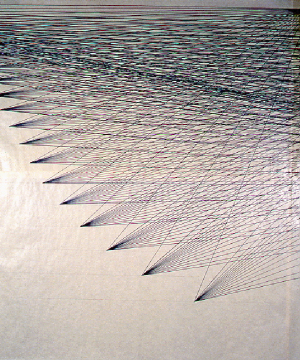An atom emitting a quantum of electromagnetic radiation is described as jumping from an upper to a lower energy level. The energy value of such a quantum equals the energy difference between the 2 levels according to equation: hT = Eu – El   (where h is Planck Constant, T is frequency).

An atom absorbing a quantum of electromagnetic radiation is described as making the inverse jump from a lower to an upper level or state. The energy value of the absorbed quantum likewise equals the energy difference between the 2 levels or states. The statement that the

'atom jumps from one level or state to another' should be seen as a METAPHOR rather than

literally taken. This is equally the case if the process is described as a 'transition' or a 'transfer' of energy between 2 levels or states. The process may also be described in terms of another metaphor. Energy level differences corresponding to frequencies of atomic line spectra may be described as frequencies at which atomic structure RESONATES. The atom may be seen as a system having a specific set of RESONANT FREQUENCIES. An incident light wave disturbs the system and causes the atom to react by radiating a wave of the same frequency as the incident wave.

These different models describing an emission-absorption process are EQUIVALENT, and should lead to equivalent artistic structures. They have a common physical skeleton expressed by the 2 basic postulates: (a) The atom is a system of ENERGY LEVELS or STATIONARY states. (b) The electromagnetic LINE SPECTRUM emitted by an atom corresponds to ENERGY DIFFERENCES  between ENERGY LEVELS OR STATES.

I applied this program to the simplest spectral set i.e. that of the Hydrogen atom (H), which represents, with its single electron, the simplest atomic structure.

3The ENERGY LEVELS of the HYDROGEN ATOM are usually represented in a DIAGRAM form after the work of Walter Grotrian. The DIAGRAM is composed of groups of PARALLEL VERTICAL LINES representing spectral lines intersecting a group of PARALLEL HORIZONTAL LINES representing energy levels. The DIAGRAM gives information about allowed energy values of different levels, relative distances between them, ionization potential, relative excitation energies,...etc. as well as the distribution of the H line spectrum into definitive spectral groups

or series. The lines of each such SERIES terminate on a specific COMMON HORIZONTAL LINE (energy level). The length of each VERTICAL (i.e. spectral) LINE connecting 2 energy levels is directly PROPORTIONAL to the FREQUENCY of the spectral line.

The DIAGRAM shows 6 spectral series:

( 1) The LYMAN series: The wavelengths of its lines fall in the ultra­violet region of the spectrum. All vertical lines representing this series terminate on the lowest level, ie. ENERGY LEVEL No.1.

(2) The BAIMER series: The wavelengths of its lines fall in the visible region. All lines representing this series terminate on ENERGY LEVEL No.2.

(3) The PASCHEN series occurs in the infra-red region. All lines of this series terminate on ENERGY LEVEL NO.3.

(4) The BRACKETT series falls in the infra-red region. All its lines terminate on ENERGY LEVEL No.4.

(5) The PFUND series falls in the infra-red region. All its lines terminate on ENERGY LEVEL No.5.

(6) Lastly the HUMPHREY series whose lines terminate on ENERGY LEVEL No.6.

4I reconstructed the foregoing spectral data according to a hypothesis allowing for a change of the diagram into a naturalistic image com­bining the colour and structural values of the H atom as a unity. The hypothesis briefly is: (1) That the single electron of the H atom is an energy concentration point of the electromagnetic field. (2) That this (electron)mass point is a curvature point of space-time.

The reconstruction proceeded as follows:

(1) In Grotrian's DIAGRAM the lengths of the VERTICAL (ie. spectral) lines connecting the HORIZONTAL (i.e. energy levels) lines are directly PROPORTIONAL to FREQUENCIES of spectral lines. I introduced a 2-FOLD CHANGE here: (a) I calculated the IENGTHS of the VERTICAL (spectral) lines in direct PROPORTION to WAVELENGTH rather than FREQUENCY.

A WAVELENGTH value is a measure of SPATIAL DISTANCE whereas FREQUENCY is a pointer of ENERGY SIZE.  As the aim is to ,construct a pictorial model representing the FORM/STRUCTURE of the R atom, WAVELENGTH rather than FREQUENCY values are relevant. (b) In addition to the WAVELENGTH value I introduced a SECOND SPATIAIL value separating the HORIZONTAL (energy levels) lines. HORIZONTAL (energy level) lines may equally be, considered as a system of concentric SHELLS whose common centre is the nucleus. Therefore their RADII values become the basis for additional DISTANCE valueso These are given by equation:

r = n2 ao   (n= 1,2,3,…etc.)

(where n is the energy-level or shell QUANTUM NUMBER, ao is Bohr radius, ie. the radius value for the first orbit of the H atom & equals 0.529 A).

Hence we have 2 DISTANCE VALUES between the HORIZONTAL (energy levels) lines representing: 1) The DISTANCE as .a RADIUS value, i.e. as a separa­tion value between electronic shells. (2) The DISTANCE as spectral wave-length value, i.e. as an electromagnetic quantum INTERVAL between 2 energy levels.

(2) I made use of the THEORETICAL POSSIBILITY which allows for an 'INFINITE' number of spectral-line series in addition to the observe~ detected spectral series. It is clear that whereas these may satisfy the requirements of a graphic diagram (such as Grotrian's) they cannot be sufficient to Build a satisfactory picture of the H atom. For this purpose it was necessary to make use of both the detected & the theo­retically possible spectral series. These are defined according to equation:

V = RH (1/n12 - 1/n22 )

where v is the wave number, RH is the Rydberg Constant for Hydrogen.

For the calculation of wave numbers of theoretically possible spectral lines and series of the H atom, we substitute the following values:

 Lyman Balmer Paschen Brackett Pfund Humphrey N1 = 1 N1 = 2 N1 = 3 N1 = 4 N1 = 5 N1 = 6 N2  >= 2 N2  >= 3 N2  >= 4 N2  >= 5 N2  >= 6 N2  >= 7

(3) The whole structure of Grotrian's DIAGRAM is one of PARALLEL lines (VERTICAL & HORIZONTAL). However, Euclid's 5th postulate of parallel lines has already been replaced by different axioms in non-Euclidean geometry. According to Riemann's amended 5th postulate 'for any line L and any point P not on L, there are no lines through P parallel to L'. Lines even seemingly parallel are bound to meet at a point. Hence a pictorial representation of a physical system such as the H atom must take this new geometrical axiom into account. A similar consideration applies on a physical level. According to Einstein's MASS-ENERGY EQUIVALENCE an elementary particle, e.g. an electron, may be postulated as a point of great concentration of energy or of field strength. Such a particle may equally be visualized as a curvature point of space-time, i.e. a cause & effect of space-time geometry curvature. This conforms to Einstein's other EQUIVALENCE principle, ie. that of PHYSICS & GEOMETRY. Both physical and geometrical considerations therefore point to a

solution favouring a principle of CONVERGENCE.

I altered Grotrian's DIAGRAM allowing for all PARALLEL lines (VERTICAL & HORIZONTAL) to converge. The VERTICAL lines of each SPECTRAL SERIES were made to converge at their specific TERMINAL (energy levels) lines thus

producing convergence points on the successive (HORIZONTAL) levels. Each such point is an energy concentration point representing a POTENTIAL electron. The HORIZONTAL (energy levels) lines were equally made to converge in proportion to the gradually decreasing wavelength values of spectral lines. All such HORIZONTAL lines finally converged forming with the converging lines of the LYMAN spectral series, an ELECTRON PARTICLE POINT on energy level No.1 (GROUND STATE).

( 4) Finally there is the question of the FINE STRUCTURE of the H spectral lines. Each energy level consists of a number of SUB-LEVELS lying very close to one another. Their number is directly proportional to the energy level quantum number. Energy differences between such SUB-LEVELS equally correspond to spectral line emissions. Consequently each H spectral line should consist of a number of components which are detectable when examined under high resolutiono The number of such fine structure lines for different spectral series is as follows:

 Lyman series Balmer series Paschen series Brackett series Pfund series Humphrey series Number of Components for each spectral line 1 3 5 7 9 11

The significance of these numbers is that they correspond to the number of SPACE ORIENTATIONS associated with the different states of motion of the electron inside the H atom. The configuration of spectral lines therefore can assume structural values corresponding to the electron states and space orientations in the various energy levels and sub­levels of the H atom. This is shown in the following table:

 SPECTRAL SERIES Lyman Balmer Paschen Brackett Pfund Humphrey ELECTRON STATES DESIGNATION s p d f g h SPACE ORIENTATION SHELL No.1 1 - - - - - SHELL No.2 1 3 - - - - SHELL No.3 1 3 5 - - - SHELL No.4 1 3 5 7 - - SHELL No.5 1 3 5 7 9 SHELL No.6 1 3 5 7 9 11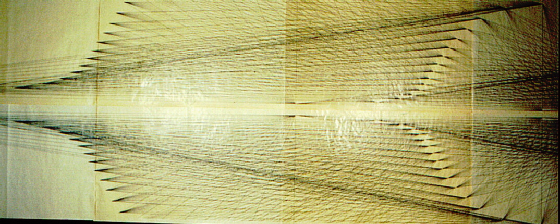Representation of the spectral lines of the H Atom - 1987

CONCLUSION:

The solution was possible by linking OBSERVABLE quantities (i.e. line spectra) with EXPERIMENTAL data (e.g. H atom structure, electron shells & states of motion,...) and THEORETICAL concepts (e.g. relativity, quantum physics ,..etc.). This made it possible to define distances & intervals and construct a 2-d. space-time metric.

The body of the H atom appears in the drawings as a complex vector space. Every theoretically possible energy transition or state of motion of the electron is described in this space by a vector representing a measurable wave-length quantity. This gives us a quantized space-time whose constituent units are measurable electromagnetic quanta. It means an EQUIVALENCE of space­-time AND electromagnetic units. But the electromagnetic field requires no supporting medium. The curved space-time IS the electromagnetic field struc­ture. And the field curvature is manifested in the different but harmoniously related wave-lengths associated with its component quanta. The dualism there­fore resolves itself by reducing space-time to measurable values of the electromagnetic field. The 'field is the only reality'.

A field quantum is characterized by a set of mathematically related values. These include VARIABLES, e.g. energy, momentum, wave-length,..., and CONSTANTS, i.e. VELOCITY c & ACTION h. However, like any other entity, a quantum has its own PROPER TIME value which may be defined as the RECIPROCAL of frequency. A. quantum therefore represents a specific 'space-time' INTERVAL, and the structure may be described in this sense as a system of such intervals.

The drawings, however, essentially reveal a curved spiral structure of energy levels (or points) and spectral lines. Zach point is a convergence point of a group of such lines. And within this structure the single electron of the H atom appears as a concentration point of the quanta of the electromagnetic (i.e. spectral) field. The electron-point is indivisible because it is the product of field curvature geometry. The field structure is the real body of the electron and extends beyond the H atom to the whole universe.

The ancients postulated LIGHT as the essential reality of the universe. Quantum Realism extends this concept to include the whole electromagnetic spectrum of which light is only a tiny region. It also identifies this spectrum with the newly discovered sub-atomic reality. The electromagnetic field is the stuff, the structure & container and the absolute messenger

of this universe.